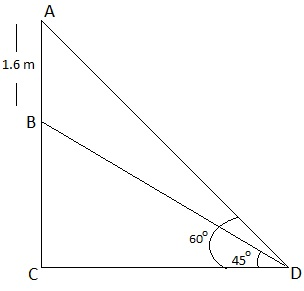Guru

# A statue, 1.6 m tall, stands on the top of a pedestal. From a point on the ground, the angle of elevation of the top of the statue is 60° and from the same point the angle of elevation of the top of the pedestal is 45°. Find the height of the pedestal. Q.8

• 0

How i solve the problem of class 10th ncert math of applications of Trigonometry chapter of exercise 9.1 of ncert of question no,8 please help me to solve this problem give me the simple and easy way to solve this problem A statue, 1.6 m tall, stands on the top of a pedestal. From a point on the ground, the angle of elevation of the top of the statue is 60° and from the same point the angle of elevation of the top of the pedestal is 45°. Find the height of the pedestal.

Share

1. Let AB be the height of statue.

D is the point on the ground from where the elevation is taken.

To Find: Height of pedestal = BC = AC-ABFrom figure,

In right triangle BCD,

tan 45° = BC/CD

1 = BC/CD

BC = CD …..(1)

Again,

In right ΔACD,

tan 60° = AC/AD

√3 = ( AB+BC)/CD

√3CD = 1.6 + BC

√3BC = 1.6 + BC (using equation (1)

√3BC – BC = 1.6

BC(√3-1) = 1.6

BC = 1.6/(√3-1) m

BC = 0.8(√3+1)

Thus, the height of the pedestal is 0.8(√3+1) m.

• 0